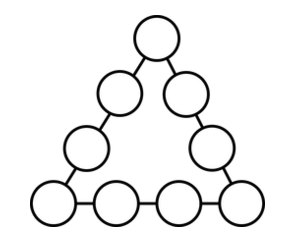# Law of Cosines Triangle

Directions: Using the digits 1 to 9 at most one time each, fill in the boxes to fill in the circles of a triangle. The sum of the numbers on each side of the triangle is equal to the length of that side. What is the triangle with the largest (or smallest) angle measure that you can make?### Hint

Be careful that you don’t make an impossible triangle! Remember the triangle inequality theorem states that the sum of the lengths of any two sides of a triangle is greater than the length of the remaining side

The smallest interior angle of a triangle is always opposite the shortest side

The largest interior angle of a triangle is always opposite the longest side

A triangle with the largest angle (there are several variations with the same angle):
Side A: 6+8+9+7=30 Side B: 7+4+1+3=15 Side C: 3+2+5+6=16
Angle A: 150.799 Angle B: 14.119 Angle C = 15.082

A triangle with the smallest angle (there are several variations with the same angle):
Side A: 1+2+3+4=​10 Side B: 4+8+9+7=28 Side C: 1+5+6+7=19
Angle A: 10.844 Angle B: 148.212 Angle C = 20.944

Source: Erick Lee

## Similar Triangles

Directions: Using the digits 0 to 9 at most one time each and as many …

1.•